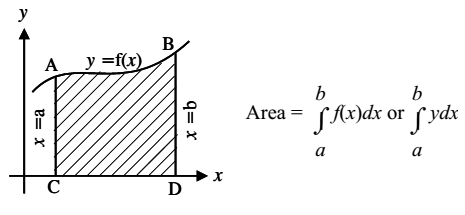# Area of Bounded Regions

Let y = f(x) be a continuous function defined on [a, b], which is positive (f(x) lies on or above x-axis) on the interval [a, b]. Then, the area bounded by the curve y = f(x), the x-axis and the ordinates x = a and x = b is given byIf f(x) ≤ 0 (f(x) lies on or below x-axis) for all x in a ≤ x ≤ b then area is given by